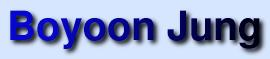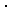Boyoon Jung's Library Manual

# bj::MRKDTree Class Reference [Machine Learning Techniques]

A multiresolution KD-Tree data structure class. More...

`#include <kd_tree.h>`

Collaboration diagram for bj::MRKDTree:[legend]
List of all members.

## Public Member Functions

MRKDTree (gsl_vector **P=0, int size=0, double mbw=-1.0)
A contructor.
~MRKDTree (void)
A destructor.
void build (gsl_vector **P, int size, double mbw=-1.0)
build a multiresolution KD-tree.
mrkd_noderoot (void)
Return the root node of a tree.
int size (void)
Return the current tree size.

## Detailed Description

A multiresolution KD-Tree data structure class.

MRTDTree class is written mainly for VFEMCluster class. For efficiency, the statistics are computed only for leaf nodes.

## Constructor & Destructor Documentation

 bj::MRKDTree::MRKDTree ( gsl_vector ** P = `0`, int size = `0`, double mbw = `-1.0` )

A contructor.

Parameters:
 P A set of data points. size The number of data points in P. mbw The minimum bounding-box width. If negative, the unit of mbw is percentage.

 bj::MRKDTree::~MRKDTree ( void )
 A destructor.

## Member Function Documentation

 void bj::MRKDTree::build ( gsl_vector ** P, int size, double mbw = `-1.0` )

build a multiresolution KD-tree.

Parameters:
 P A set of data points. size The number of data points in P. mbw The minimum bounding-box width. If negative, the unit of mbw is percentage.

 mrkd_node* bj::MRKDTree::root ( void ) ` [inline]`
 Return the root node of a tree.

 int bj::MRKDTree::size ( void ) ` [inline]`
 Return the current tree size.

The documentation for this class was generated from the following file:
ResearchProjectsPublications

ToolsBjLib ManualPlayer/StagePyPlayerMicroStrain IMUGPS/DGPSParticle Filter

PersonalWelcomeCurriculum VitaeResumeContact

Outside LinksUSCUSC CS DeptCRESRobotics LabRESL